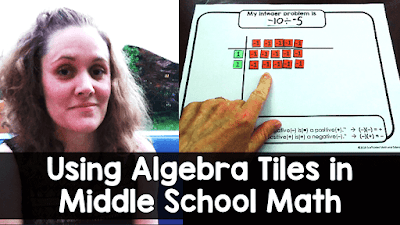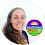## Pages

### Solving equations using algebra tiles: with pictures!

There are so many cool ways to use algebra tiles in math. Last week I wrote a post about using them to factor. In this post I wanted to show 3 examples for using algebra tiles to solve equations.

New to algebra tiles?

I'm using a free set of paper algebra tiles (linked below), mainly so that I could cut one in half for example 2 that involves a fraction. So let's get into it!

Example 1:

Solve 2x + 3 = 11 with algebra tiles.

>>A paper version of the solving mat can be found for free here or

The rectangular tiles are used to represent x and the small squares are each used to represent 1.

In this photo, the 3 squares on the left got recycled along with 3 squares on the right, leaving 2x = 8.

Here's the fun part! We can split the 2x into x and x and make equal groups of the small squares on the other side.

After recycling one group, we get x = 4.

Using algebra tiles to solve 2-step equations is an incredible way to introduce the topic.

Example 2:
Solve ½x + 4 = 7 with algebra tiles.

It's nice to use paper algebra tiles for this example so that an x can be cut in half. (We still keep the other half for the end.)

First I cut an x in half to show the ½x.

Here we can see our equation set up.

We recycle the 4 squares on the left and 4 on the right, leaving ½x = 3.

Now we bring in that other ½x from earlier. Because we just doubled our x, we have to double the other side. This gives us x = 6.

Example 3:

Solve 2x - 3 = 7 with algebra tiles.

To get negative tiles with paper, you can print on 2-sided paper, like astrobrights, or glue two pieces of paper together before cutting.

Here we have 2 x tiles and 3 negative tiles on the left and 7 tiles on the right.

To get rid of that -3 on the left, we +3 to make a zero pair. Then we have to +3 to the right, too.

The zero pair gets recycled since it's 0. Now we have 2x = 10.

And now back to the fun part: splitting into equal groups.

And recycling the extra stuff to get x = 5.

Video:
Here is a video showing all 3 examples in this post:

UPDATE: I made a set of digital algebra tiles in Google Slides that are set up for solving equations. They are linked below:

Resources:

Algebra tiles can be found here.

Paper solving mat can be found here.

NEW! Digital algebra tiles for solving equations can be found here.

Solving equations activities can be found here.

If you'd like to learn more about ways to use algebra tiles, I have put together an algebra tiles tutorial video that covers ways to use algebra tiles in middle school math.

### Using Algebra Tiles in Middle School Math:watch algebra tiles tutorial video w/ free algebra tiles

1.AnonymousJune 15, 2022

Looks like your "Digital Google Slides solving mat" link has been hacked. Everytime I click on it, it brings me to corrupt site.

1.Thank you so much for letting me know. That was a link to a friend's blog. I will unlink that text. I really appreciate the help.

2.UPDATE: I made a Google Slides set of algebra tiles specific to solving that is now linked in the post.

2.Hi There. I found the template for the solving equations algebra tiles mat (that you can laminate and wipe clean). Do you have any similar resources for factorising, expanding, combining like terms etc?

1.Hi Jemma, I do have a pack of them linked in my algebra tiles video tutorial post here: https://www.scaffoldedmath.com/p/algebra-tiles-tutorial.html

Comment